Updating search results...

# 35 Results

View
Selected filters:
• CalculusConditional Remix & Share Permitted
CC BY-NC-SA
Rating
0.0 stars

Some of the topics that this book addresses are: Vector spaces; finite-dimensional vector spaces; differential calculus; compactness and completeness; scalar product space; differential equations; multilenear functionals; integration; differentiable manifolds; integral calculus on manifolds; exterior calculus.

Note: this is a 57 MB PDF Document.

Subject:
Calculus
Mathematics
Material Type:
Textbook
Provider:
Harvard University
Provider Set:
Individual Authors
Author:
Sternberg Shlomo and Lynn Loomis
10/13/2017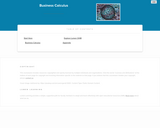Unrestricted Use
CC BY
Rating
0.0 stars

This course provides an introduction to applied concepts in Calculus that are relevant to the managerial, life, and social sciences. Students should have a firm grasp of the concept of functions to succeed in this course. Topics covered include derivatives of basic functions and how they can be used to optimize quantities such as profit and revenues, as well as integrals of basic functions and how they can be used to describe the total change in a quantity over time.

Subject:
Calculus
Mathematics
Material Type:
Full Course
Textbook
Provider:
Lumen Learning
Provider Set:
Candela Courseware
10/13/2017Unrestricted Use
CC BY
Rating
0.0 stars

MATH&148 is a calculus course for business students. It is designed for students who want a brief course in calculus. Topics include differential and integral calculus of elementary functions. Problems emphasize business and social science applications. Translating words into mathematics and solving word problems are emphasized over algebra. Applications are mainly business oriented (e.g. cost, revenue, and profit). Mathematical theory and complex algebraic manipulations are not mainstays of this course, which is designed to be less rigorous than the calculus sequence for scientists and engineers. Topics are presented according to the rule of four: geometrically, numerically, analytically, and verbally. That is, symbolic manipulation must be balanced with graphical interpretation, numerical examples, and writing. Trigonometry is not part of the course.

Subject:
Calculus
Mathematics
Material Type:
Assessment
Full Course
Syllabus
Textbook
Provider:
Washington State Board for Community & Technical Colleges
Provider Set:
Open Course Library
10/13/2017Conditional Remix & Share Permitted
CC BY-NC-SA
Rating
0.0 stars

Published in 1991 by Wellesley-Cambridge Press, the book is a useful resource for educators and self-learners alike. It is well organized, covers single variable and multivariable calculus in depth, and is rich with applications.

In addition to the Textbook, there is also an online Instructor's Manual and a student Study Guide. Prof. Strang has also developed a related series of videos, Highlights of Calculus, on the basic ideas of calculus.

Subject:
Calculus
Mathematics
Material Type:
Full Course
Provider:
M.I.T.
Author:
Gilbert Strang
01/01/1991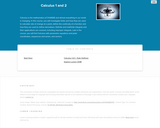Unrestricted Use
CC BY
Rating
0.0 stars

Calculus is the mathematics of CHANGE and almost everything in our world is changing. In this course, you will investigate limits and how they are used to calculate rate of change at a point, define the continuity of a function and how they are used to define derivatives. Definite and indefinite integrals and their applications are covered, including improper integrals. Late in the course, you will find Calculus with parametric equations and polar coordinates, sequences and series, and vectors.

Subject:
Calculus
Mathematics
Material Type:
Full Course
Textbook
Provider:
Lumen Learning
Provider Set:
Candela Courseware
Author:
Dale Hoffman
David Lippman
Jeff Eldridge
Melonie Rasmussen
10/13/2017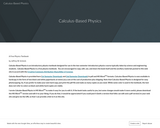Conditional Remix & Share Permitted
CC BY-SA
Rating
0.0 stars

Calculus-Based Physics is an introductory physics textbook designed for use in the two-semester introductory physics course typically taken by science and engineering students.

Subject:
Calculus
Mathematics
Physical Science
Physics
Material Type:
Textbook
Provider:
Saint Anselm College
Author:
Jeffrey
Schnick
11/09/2017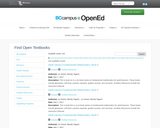Unrestricted Use
CC BY
Rating
0.0 stars

Calculus-Based Physics is an introductory physics textbook designed for use in the two-semester introductory physics course typically taken by science and engineering students

Subject:
Calculus
Mathematics
Physical Science
Physics
Material Type:
Textbook
Provider:
BCcampus
Provider Set:
BCcampus Open Textbooks
Author:
Jeffrey W. Schnick
10/28/2014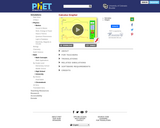Unrestricted Use
CC BY
Rating
0.0 stars

Draw a graph of any function and see graphs of its derivative and integral. Don't forget to use the magnify/demagnify controls on the y-axis to adjust the scale.

Subject:
Calculus
Mathematics
Material Type:
Simulation
Provider:
Provider Set:
PhET Interactive Simulations
Author:
Dubson, Michael
Loeblein, Trish
Michael Dubson
PhET Interactive Simulations
Trish Loeblein
08/31/2009Unrestricted Use
CC BY
Rating
0.0 stars

Draw a graph of any function and see graphs of its derivative and integral. Don't forget to use the magnify/demagnify controls on the y-axis to adjust the scale.

Subject:
Calculus
Mathematics
Material Type:
Simulation
Provider:
Provider Set:
PhET Interactive Simulations
Author:
Dubson, Michael
Loeblein, Trish
02/02/2013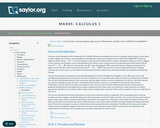Unrestricted Use
CC BY
Rating
0.0 stars

This course begins with a review of algebra specifically designed to help and prepare the student for the study of calculus, and continues with discussion of functions, graphs, limits, continuity, and derivatives. The appendix provides a large collection of reference facts, geometry, and trigonometry that will assist in solving calculus problems long after the course is over. Upon successful completion of this course, the student will be able to: calculate or estimate limits of functions given by formulas, graphs, or tables by using properties of limits and LĺÎĺ_ĺĚĺ_hopitalĺÎĺ_ĺĚĺ_s Rule; state whether a function given by a graph or formula is continuous or differentiable at a given point or on a given interval and justify the answer; calculate average and instantaneous rates of change in context, and state the meaning and units of the derivative for functions given graphically; calculate derivatives of polynomial, rational, common transcendental functions, and implicitly defined functions; apply the ideas and techniques of derivatives to solve maximum and minimum problems and related rate problems, and calculate slopes and rates for function given as parametric equations; find extreme values of modeling functions given by formulas or graphs; predict, construct, and interpret the shapes of graphs; solve equations using NewtonĺÎĺ_ĺĚĺ_s Method; find linear approximations to functions using differentials; festate in words the meanings of the solutions to applied problems, attaching the appropriate units to an answer; state which parts of a mathematical statement are assumptions, such as hypotheses, and which parts are conclusions. This free course may be completed online at any time. It has been developed through a partnership with the Washington State Board for Community and Technical Colleges; the Saylor Foundation has modified some WSBCTC materials. (Mathematics 005)

Subject:
Calculus
Functions
Mathematics
Material Type:
Assessment
Full Course
Syllabus
Textbook
Provider:
The Saylor Foundation
10/13/2017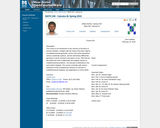Conditional Remix & Share Permitted
CC BY-NC-SA
Rating
0.0 stars

This course is an introduction to the calculus of functions of several variables. It begins with studying the basic objects of multidimensional geometry: vectors and vector operations, lines, planes, cylinders, quadric surfaces, and various coordinate systems. It continues with the elementary differential geometry of vector functions and space curves. After this, it extends the basic tools of differential calculus - limits, continuity, derivatives, linearization, and optimization - to multidimensional problems. The course will conclude with a study of integration in higher dimensions, culminating in a multidimensional version of the substitution rule.

Subject:
Calculus
Geometry
Mathematics
Material Type:
Full Course
Homework/Assignment
Syllabus
Provider:
UMass Boston
Provider Set:
UMass Boston OpenCourseWare
Author:
Catalin Zara
Ph.D.
10/13/2017Unrestricted Use
CC BY
Rating
0.0 stars

This contemporary calculus course is the third in a three-part sequence. In this course students continue to explore the concepts, applications, and techniques of Calculus - the mathematics of change. Calculus has wide-spread application in science, economics and engineering, and is a foundation college course for further work in these areas. This is a required class for most science and mathematics majors.Login: guest_oclPassword: ocl

Subject:
Calculus
Mathematics
Material Type:
Full Course
Homework/Assignment
Lecture Notes
Lesson Plan
Syllabus
Provider:
Washington State Board for Community & Technical Colleges
Provider Set:
Open Course Library
10/31/2011Unrestricted Use
CC BY
Rating
0.0 stars

This contemporary calculus course is the second in a three-part sequence. In this course students continue to explore the concepts, applications, and techniques of Calculus - the mathematics of change. Calculus has wide-spread application in science, economics and engineering, and is a foundation college course for further work in these areas. This is a required class for most science and mathematics majors.Login: guest_oclPassword: ocl

Subject:
Calculus
Mathematics
Material Type:
Full Course
Homework/Assignment
Lecture Notes
Lesson Plan
Syllabus
Provider:
Washington State Board for Community & Technical Colleges
Provider Set:
Open Course Library
10/31/2011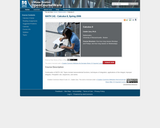Conditional Remix & Share Permitted
CC BY-NC-SA
Rating
0.0 stars

Topics in this course include transcendental functions, techniques of integration, applications of the integral, improper integrals, l'Hospital's rule, sequences, and series.

Subject:
Calculus
Mathematics
Material Type:
Full Course
Homework/Assignment
Syllabus
Provider:
UMass Boston
Provider Set:
UMass Boston OpenCourseWare
Author:
Catalin Zara
Ph.D.
10/13/2017Unrestricted Use
CC BY
Rating
0.0 stars

This course is an introduction to contemporary calculus and is the first of a three-part sequence. In this course students explore the concepts, applications, and techniques of Calculus - the mathematics of change. Calculus has wide-spread application in science, economics and engineering, and is a foundation college course for further work in these areas. This is a required class for most science and mathematics majors.Login: guest_oclPassword: ocl

Subject:
Calculus
Functions
Mathematics
Material Type:
Full Course
Homework/Assignment
Lecture Notes
Lesson Plan
Syllabus
Provider:
Washington State Board for Community & Technical Colleges
Provider Set:
Open Course Library
10/31/2011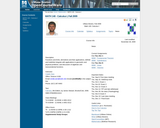Conditional Remix & Share Permitted
CC BY-NC-SA
Rating
0.0 stars

This course is an introduction to differential and integral calculus. It begins with a short review of basic concepts surrounding the notion of a function. Then it introduces the important concept of the limit of a function, and use it to study continuity and the tangent problem. The solution to the tangent problem leads to the study of derivatives and their applications. Then it considers the area problem and its solution, the definite integral. The course concludes with the calculus of elementary transcendental functions.

Subject:
Calculus
Mathematics
Material Type:
Full Course
Homework/Assignment
Syllabus
Provider:
UMass Boston
Provider Set:
UMass Boston OpenCourseWare
Author:
Catalin Zara
10/13/2017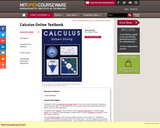Conditional Remix & Share Permitted
CC BY-NC-SA
Rating
0.0 stars

Published in 1991 and still in print from Wellesley-Cambridge Press, the book is a useful resource for educators and self-learners alike. It is well organized, covers single variable and multivariable calculus in depth, and is rich with applications. There is also an online Instructor's Manual and a student Study Guide. Prof. Strang has also developed a related series of videos, Highlights of Calculus, on the basic ideas of calculus.

Subject:
Calculus
Mathematics
Material Type:
Full Course
Provider:
M.I.T.
Provider Set:
M.I.T. OpenCourseWare
Author:
Strang, Gilbert
01/01/2005Conditional Remix & Share Permitted
CC BY-NC-SA
Rating
0.0 stars

Calculus Revisited is a series of videos and related resources that covers the materials normally found in a freshman-level introductory calculus course.&nbsp; The series was first released in 1970 as a way for people to review the essentials of calculus.&nbsp; It is equally valuable for students who are learning calculus for the first time.

Subject:
Calculus
Mathematics
Material Type:
Full Course
Provider:
M.I.T.
Provider Set:
M.I.T. OpenCourseWare
Author:
Gross, Herbert
11/18/2012Conditional Remix & Share Permitted
CC BY-NC-SA
Rating
0.0 stars

Calculus Revisited is a series of videos and related resources that covers the materials normally found in freshman- and sophomore-level introductory mathematics courses. Multivariable Calculus is the second course in the series, consisting of 26 videos, 4 Study Guides, and a set of Supplementary Notes. The series was first released in 1971 as a way for people to review the essentials of calculus. It is equally valuable for students who are learning calculus for the first time.

Subject:
Calculus
Mathematics
Material Type:
Full Course
Provider:
M.I.T.
Provider Set:
M.I.T. OpenCourseWare
Author:
Herbert Gross
01/01/2011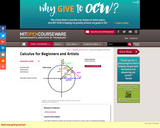Conditional Remix & Share Permitted
CC BY-NC-SA
Rating
0.0 stars

This online textbook provides an overview of Calculus in clear, easy to understand language designed for the non-mathematician.

Subject:
Calculus
Mathematics
Material Type:
Full Course
Provider:
M.I.T.
Provider Set:
M.I.T. OpenCourseWare
Author:
Kleitman, Daniel